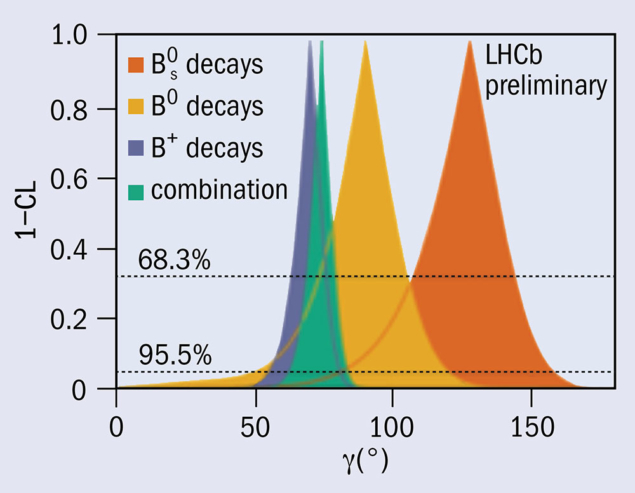# LHCb tests consistency of unitarity triangle

31 August 2018Constraints on the unitarity-triangle angle γ from LHCb measurements grouped by the type of B meson considered, along with the overall combination. The y-axis shows (1−CL), where CL is the confidence level.

A report from the LHCb experiment

Since the beginning of the LHC physics programme in 2010, the LHCb collaboration has been working to drive down the uncertainty on the least-precisely measured angle of the unitarity triangle, γ. The unitarity triangle exists in the complex plane and its area is a measure of the amount of CP violation in the Standard Model. Mathematically, the triangle represents a requirement that the Cabibbo–Kobayashi–Maskawa (CKM) quark-mixing matrix is unitary, meaning that the number of quarks is conserved in weak interactions and that there are only three generations of quarks. If new physics exists and breaks these assumptions, it would show up as internal inconsistencies in the unitarity triangle – for example, the angles of the triangle might not add up to 180°. Checking the consistency of different measurements of the unitarity triangle is therefore an important test of the SM.

The unitarity triangle exists in the complex plane and its area is a measure of the amount of CP violation in the Standard Model.

Experimentally, γ can be measured through the interference between b̅ c̅ u s̅ and b̅ u̅ c s̅ transitions. It is the only CKM angle that is easily accessible in tree-level processes and, as a result, it can be measured with negligible theoretical uncertainty. In the absence of new-physics effects at tree level, a precise measurement of γ can be compared with other observables related to the CKM matrix that are more likely to be affected by physics beyond the SM. Such comparisons are currently limited by the relatively large uncertainty on γ.

LHCb has recently made a model-independent study of the decay mode B± DK± (where D could be D0 or D̅0), with the D meson being reconstructed via the decays D KS0 π+ π and D KS0 K+ K. This measurement is particularly important for determining γ, as it selects a single solution without ambiguities and with small uncertainty. Because the D meson undergoes a three-body decay, the distribution of events across the phase space (the Dalitz plot) carries information about the underlying amplitudes. And since the B± DK± amplitudes depend on γ, it is possible to measure γ by comparing the distributions for B+ and B. In practice, the distributions depend mainly on the amplitudes of the D decay, with only small CP-violating deviations introduced by γ. The measurement therefore demands a good understanding of the magnitudes of the D0 and D̅0 decay amplitudes, as well as the strong phase differences between them, δD. The former comes from high-statistics calibration channels, and the latter from external measurements performed by the CLEO collaboration.

The new measurement uses 2 fb−1 of proton–proton collision data taken in 2015 and 2016, with signals of about 4100 B± D K± decays for the more copious D KS0 π+ π mode and about 560 for D KS0 K+ K. LHCb found γ = 87+11–12 °, which is consistent with the previous world average, as well as measuring other decay parameters rB = 0.087+0.013–0.014  and δB = 101±11°. This is the most precise determination of γ from a single analysis, and LHCb has performed several other measurements of γ, each providing different constraints. Their combination (γ = 74.0+5.0–5.8°, see figure) dominates the current world average and allows increasingly precise tests for new physics by probing the internal consistency of the unitarity triangle.